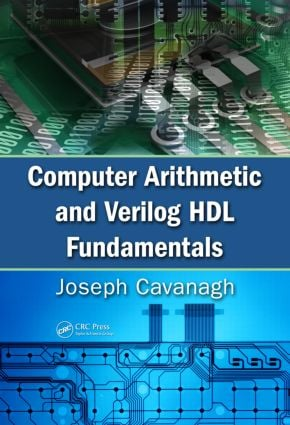# Computer Arithmetic and Verilog HDL Fundamentals

## 1st Edition

CRC Press

971 pages | 750 B/W Illus.

Hardback: 9781439811245
pub: 2009-11-24
\$195.00
x
eBook (VitalSource) : 9781315218229
pub: 2017-12-19
from \$117.00

FREE Standard Shipping!

### Description

Verilog Hardware Description Language (HDL) is the state-of-the-art method for designing digital and computer systems. Ideally suited to describe both combinational and clocked sequential arithmetic circuits, Verilog facilitates a clear relationship between the language syntax and the physical hardware. It provides a very easy-to-learn and practical means to model a digital system at many levels of abstraction.

Computer Arithmetic and Verilog HDL Fundamentals details the steps needed to master computer arithmetic for fixed-point, decimal, and floating-point number representations for all primary operations. Silvaco International’s SILOS, the Verilog simulator used in these pages, is simple to understand, yet powerful enough for any application. It encourages users to quickly prototype and de-bug any logic function and enables single-stepping through the Verilog source code. It also presents drag-and-drop abilities.

Introducing the three main modeling methods—dataflow, behavioral, and structural—this self-contained tutorial—

• Covers the number systems of different radices, such as octal, decimal, hexadecimal, and binary-coded variations
• Reviews logic design fundamentals, including Boolean algebra and minimization techniques for switching functions
• Presents basic methods for fixed-point addition, subtraction, multiplication, and division, including the use of decimals in all four operations
• Addresses floating-point addition and subtraction with several numerical examples and flowcharts that graphically illustrate steps required for true addition and subtraction for floating-point operands
• Demonstrates floating-point division, including the generation of a zero-biased exponent

Designed for electrical and computer engineers and computer scientists, this book leaves nothing unfinished, carrying design examples through to completion. The goal is practical proficiency. To this end, each chapter includes problems of varying complexity to be designed by the reader.

### Reviews

"Cavanagh has provided readers with a very large work on the topics of addition, subtraction, multiplication, and division. … The student who completes a course based on this work will have achieved a great deal. Summing Up: Recommended."

CHOICE, June 2010

Chapter 1 Number Systems and Number Representations

Number Systems

Number Representations

Chapter 2 Logic Design Fundamentals

Boolean Algebra

Minimization Techniques

Combinational Logic

Sequential Logic

Chapter 3 Introduction to Verilog HDL

Built-In Primitives

User-Defined Primitives

Dataflow Modeling

Behavioral Modeling

Structural Modeling

Chapter 5 Fixed-Point Subtraction

Twos Complement Subtraction

Ripple-Carry Subtraction

Chapter 6 Fixed-Point Multiplication

Booth Algorithm Multiplication

Bit-Pair Recoding Multiplication

Array Multiplication

Table Lookup Multiplication

Memory-Based Multiplication

Multiple-Operand Multiplication

Chapter 7 Fixed-Point Division

SRT Division

Multiplicative Division

Array Division

Chapter 9 Decimal Subtraction

Subtraction Examples

Chapter 10 Decimal Multiplication

Binary-to-BCD Conversion

Multiplication Using Behavioral Modeling

Multiplication Using Structural Modeling

Multiplication Using Memory

Multiplication Using Table Lookup

Chapter 11 Decimal Division

Restoring Division — Version 1

Restoring Division — Version 2

Division Using Table Lookup

Floating-Point Format

Biased Exponents

Overflow and Underflow

General Floating-Point Organization

Verilog HDL Implementation

Chapter 13 Floating-Point Subtraction

Numerical Examples

Flowcharts

Verilog HDL Implementations

Chapter 14 Floating-Point Multiplication

Double Bias

Flowcharts

Numerical Examples

Verilog HDL Implementations

Chapter 15 Floating-Point Division

Zero Bias

Exponent Overflow/Underflow

Flowcharts

Numerical Examples

Rounding Methods

Guard Bits

Verilog HDL Implementations

Chapter 17 Additional Topics in Computer Arithmetic

Residue Checking

Parity-Checked Shift Register

Parity Prediction

Logical and Algebraic Shifters

Arithmetic and Logic Units

Count-Down Counter

Shift Registers

Appendices

Index